Courses

# Test: Give And Take - 1

## 10 Questions MCQ Test Mathematics (Maths) for Class 2 | Test: Give And Take - 1

Description
This mock test of Test: Give And Take - 1 for Class 2 helps you for every Class 2 entrance exam. This contains 10 Multiple Choice Questions for Class 2 Test: Give And Take - 1 (mcq) to study with solutions a complete question bank. The solved questions answers in this Test: Give And Take - 1 quiz give you a good mix of easy questions and tough questions. Class 2 students definitely take this Test: Give And Take - 1 exercise for a better result in the exam. You can find other Test: Give And Take - 1 extra questions, long questions & short questions for Class 2 on EduRev as well by searching above.
QUESTION: 1

### After spending Rs. 260, Harsha had Rs. 300 left. How much did he have at first?

Solution:

Rs. 260 + Rs. 300 = Rs. 560

QUESTION: 2

### Identify the missing number in the given triangle.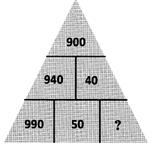Solution:

900 = 940 - 40 and
940 = 990 - 50
∴ 40 = 50 - 10

QUESTION: 3

### What are the missing numbers?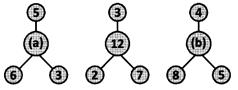Solution:

+ 7 + 3 = 12 and 3 + 1 + 5 = 9.
So, a = 5 + 6 + 3 = 14 and b = 4 + 8 + 5 = 17.

QUESTION: 4

I am a 2-digit number. The sum of my 2 digits is 9. Their difference is 1-The larger digit is in the tens place. Who am I?

Solution:

The sum of the digits in 54, is 5 + 4 = 9.
The difference of the digits in 54 is 5 - 4 =1.
The larger digit is in the tens place.
So, the required two digit number is 54.

QUESTION: 5

By how much is 14, more than 10 and by how much is it less than 24?

Solution:
QUESTION: 6

Sita collected 630 stamps. She gave 50 stamps to Rama. How many stamps are remaining with Sita?

Solution:

630-50=580

QUESTION: 7

To balance the see-saw using the five numbers given, the sum of the numbers on each side of the see-saw must be equal.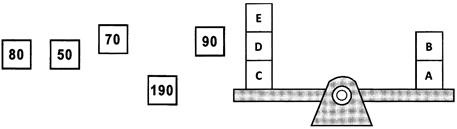What is the value of A if A is greater than B?

Solution:

A + 8 = 190 + 50 = 240
C + D + E = 70 + 80 + 90 = 240
So, A = 190.

QUESTION: 8

2 tens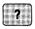ones + 4 tens 6 ones = 81. Find the number that should be placed in the place of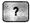.

Solution:

15 ones as 35 + 46 = 81

QUESTION: 9

Which of the following gives the greatest odd number as the answer?

Solution:
QUESTION: 10

?I am more than 2 + 4 but less than 10 - 2. Who am I??

Solution: